Question

# Complete the following table for the simple discount notes. Use the ordinary interest method. (Use 360...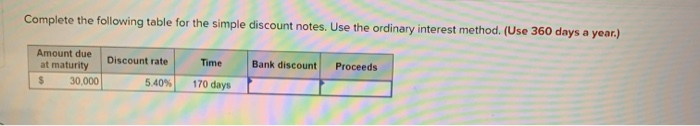Complete the following table for the simple discount notes. Use the ordinary interest method. (Use 360 days a year.) Bank discount Proceeds Amount due at maturity 30.000 Discount rate 5.40% Time 170 days

Bank discount = Amount due at maturity * Discount rate * Number of years
= \$30,000 * 5.40% * (170/360)
= \$765

Proceeds = Amount due at maturity - Bank discount
= \$30,000 - \$765
= \$29,235

#### Earn Coins

Coins can be redeemed for fabulous gifts.

Similar Homework Help Questions
• ### The face value of a simple discount note is \$4,000. The bank discount is calculated at...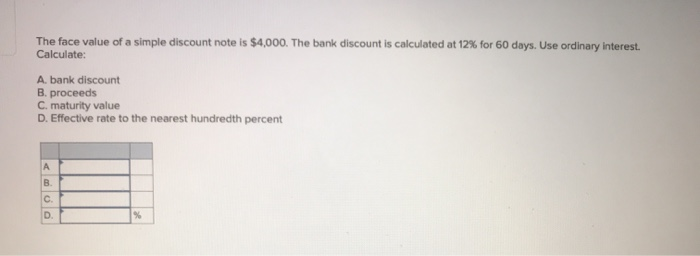The face value of a simple discount note is \$4,000. The bank discount is calculated at 12% for 60 days. Use ordinary interest. Calculate: A bank discount B. proceeds C. maturity value D. Effective rate to the nearest hundredth percent

• ### Solve for maturity value, discount period, bank discount, and proceeds.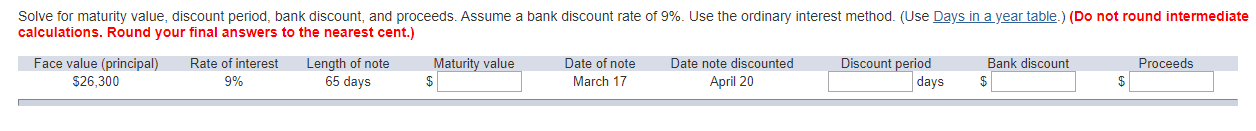Solve for maturity value, discount period, bank discount, and proceeds. Assume a bank discount rate of 9%. Use the ordinary interest method. (Use Days in a year table.) (Do not round intermediate calculations. Round your final answers to the nearest cent.)

• ### Please show work :) Complete the following using ordinary interest. (Use Days in a year table.)...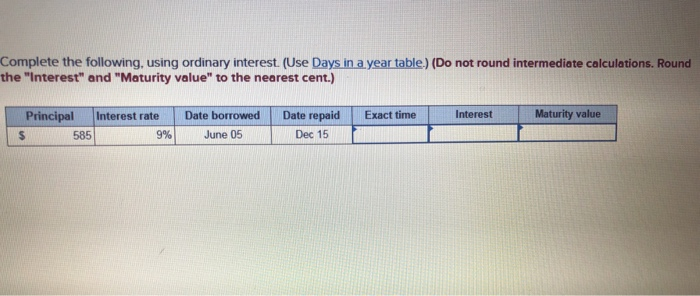Please show work :) Complete the following using ordinary interest. (Use Days in a year table.) (Do not round intermediate calculations. Round the "Interest" and "Maturity value to the nearest cent.) Exact time Interest Maturity value Principal Interest rate \$ 585 9% Date borrowed June 05 Date repaid Dec 15

• ### Bill Blank signed an \$7,540 note at Citizen's Bank. Citizen's charges a 8.2% discount rate. Assume...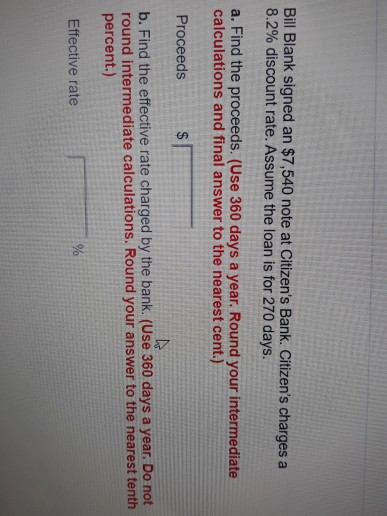Bill Blank signed an \$7,540 note at Citizen's Bank. Citizen's charges a 8.2% discount rate. Assume the loan is for 270 days. a. Find the proceeds. (Use 360 days a year. Round your intermediate calculations and final answer to the nearest cent.) Proceeds b. Find the effective rate charged by the bank. (Use 360 days a year. Do not round intermediate calculations. Round your answer to the nearest tenth percent.) Effective rate You were offered the opportunity to purchase either...

• ### A man has a simple discount note for ​\$6,200​, at an ordinary bank discount rate of...

A man has a simple discount note for ​\$6,200​, at an ordinary bank discount rate of 8.53%, for 50 days. What is the effective interest​ rate using the banker's rule?

• ### Simple Interest USE A 360 DAY YEAR Calculate the simple interest amount and the future value...

Simple Interest USE A 360 DAY YEAR Calculate the simple interest amount and the future value using the simple interest formula.                                                                                  365 day year Principal Interest Rate Time Simple Interest Amount Future Value \$ 18,000 4.5% 18 months \$ 21,000 5% 1.75 Years \$ 18,000 7.25% 9 months \$ 1,000 8% 93 days \$ 585 9% 193 days \$ 1,200 12% 187 days 1) Leslie Hart borrowed \$15,000 to pay for her child’s education. Leslie must repay the loan...

• ### Complete the table to calculate the interest amounts and use those calculated values to prepare your...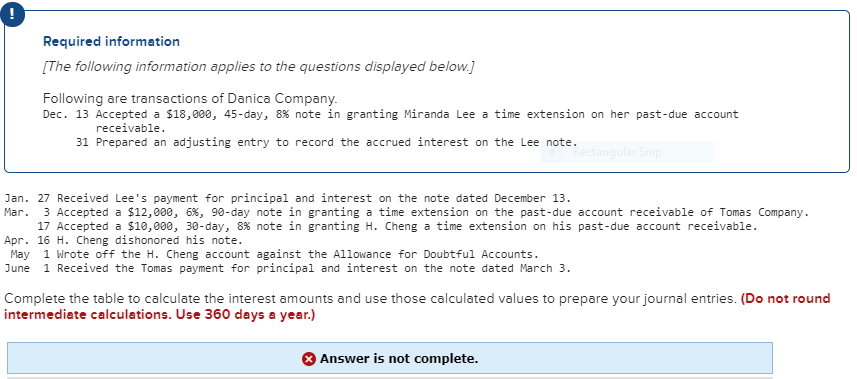Complete the table to calculate the interest amounts and use those calculated values to prepare your journal entries. I already filled out the other charts. It still says "not complete" so I must be missing a tab in the journals. I need help filling this chart out. I typed 0 as place keepers. Required information [The following information applies to the questions displayed below.] Following are transactions of Danica Company. Dec. 13 Accepted a \$18,000, 45-day, 8% note in granting...

• ### Compute interest and find the maturity date for the following notes. (Use 360 days for calculation.)...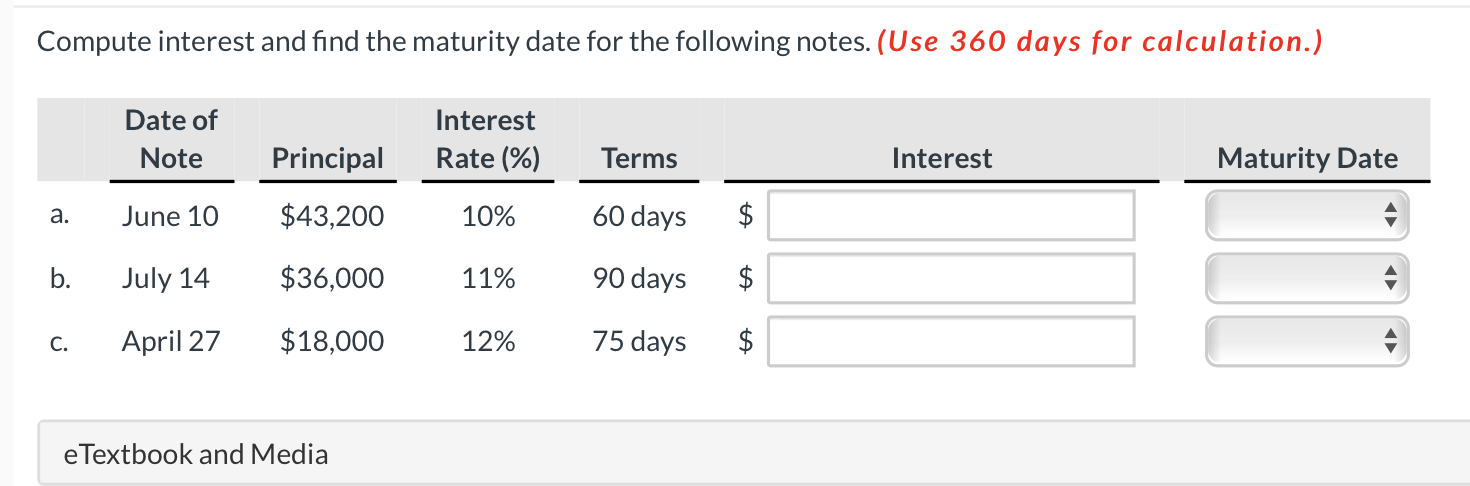Compute interest and find the maturity date for the following notes. (Use 360 days for calculation.) Date of Note Interest Rate (%) Principal Terms Interest Maturity Date a. June 10 \$43,200 10% 60 days \$ b. July 14 \$36,000 11% 90 days \$ C. April 27 \$18,000 12% 75 days \$ \$ e Textbook and Media

• ### Use ordinary interest: Principal \$70,000 Rate of Interest: 11% Time: 90 days Maturity Value: A Date note made Mar 10 Date note discounted: April 15 discount period: B proceeds: C note to be discounted at 10%

Use ordinary interest: Principal \$70,000 Rate of Interest: 11% Time: 90 days Maturity Value: A Date note made Mar 10 Date note discounted: April 15 discount period: B proceeds: C note to be discounted at 10%

• ### For each of the following notes receivable, compute the amount of interest revenue eamed during 2018. Use a 360-da...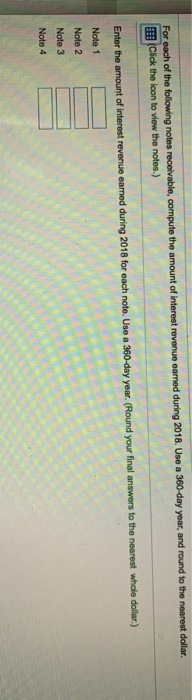For each of the following notes receivable, compute the amount of interest revenue eamed during 2018. Use a 360-day year, and round to the nearest dollar Click the icon to view the notes.) Enter the amount of interest revenue earned during 2018 for each note. Use a 360-day year. (Round your final answers to the nearest whole dollar.) Note 1 Note 2 Note 3 Note 4 i Data Table Interest Period During 2018 2 months Principal Interest Rate Note 1...